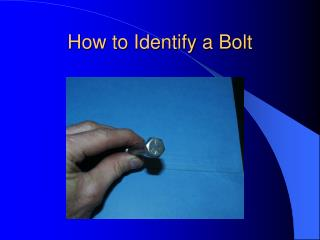DownloadDownload PresentationHow to Identify a Bolt

# How to Identify a Bolt

Télécharger la présentation## How to Identify a Bolt

- - - - - - - - - - - - - - - - - - - - - - - - - - - E N D - - - - - - - - - - - - - - - - - - - - - - - - - - -
##### Presentation Transcript

1. How to Identify a Bolt

2. The parts of a Bolt

3. One Side is Inches and the other is Millimeters

4. The 16th Scale is easier than the 32nd Scale for measuring Fractional bolts.

5. Step 1 • Determine if the bolt is Metric or Standard

6. Is this metric or standard

7. Is this metric or standard?

8. Step 2 is to determine the Size • Size means the diameter of the bolt across the threads.

9. What is the size of this standard bolt?

10. What is the size of this metric bolt?

11. Step 3 is to determine the thread pitch. • Pitch means the number of threads per inch for a standard bolt. • Pitch means the distance between threads for a metric bolt.

13. What is the pitch of this metric bolt?

14. What is the pitch of this standard bolt?

15. Step 4 is to measure the length • Length is measured from the bottom of the head to the end of the thread

16. What is the length of this standard bolt?

17. What is the length of this metric bolt?

18. The 5th step is to determine the Head size. • Head size is measured from flat to flat.

19. What is the head size of this metric bolt?

20. What is the head size of this standard bolt?

21. If the threads are damaged what tool is used to repair them?

22. Die

23. Die Size • Standard Die Size – Pitch Example: 3/8 – 14 Metric Die Size – Pitch Example: 10 X 1.25